• Hash256
• Hash160
• Reverse Bytes
• Satoshis

# Digital Signatures (Signing & Verifying)

Proving that a signature and public key were created by the same private key.

## Signing

A digital signature has two parts:

1. A random part.
2. A signature part (`private key` + `the transaction data we’re creating the digital signature for`).

### 1. Random Part

Start by generating a random number. Then multiply this with the generator point on the elliptic curve (the same generator point used when making public keys):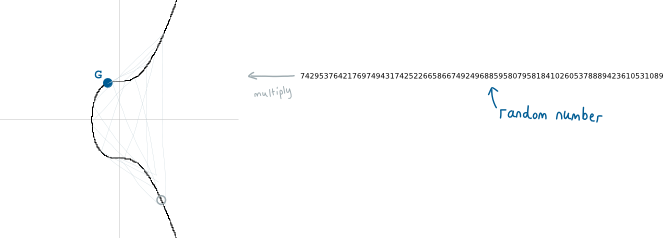The random part of our digital signature is the point on the curve that we end up with. But we’ll just take the x-coordinate of it: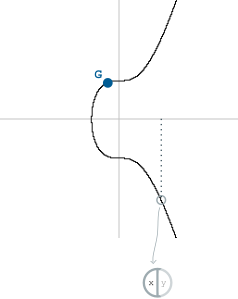We’ll call this “r” for short.This is basically the same thing as creating a private key and a public key. But here we’re doing it to add a random element to our digital signature.

So now we’ve got half of our digital signature ready, but we haven’t used our private key for anything yet. This is where the second half comes in…

### 2. Signature Part

First we take our private key, and multiply it with `r` (the x-coordinate of that random point on the curve we just found).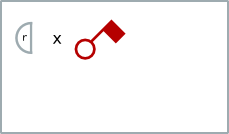Next we want to include the thing we want to sign. This is called the `message`. In bitcoin, the `message` is the hash of the entire transaction data that contains the output that we want to unlock.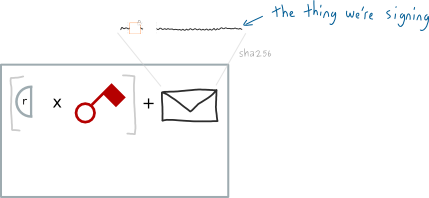Including transaction hash ties the signature to one transaction (so it can’t be used within a different transaction).

Finally, for good measure, we divide all of this by that initial random number we started with: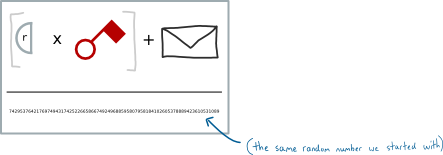And hey presto, we have the vital “signature” part of our digital signature. We’ll call this `s` for short.

Now here’s the fun bit…

If someone asks us to prove that we know the private key for a public key, we can give them our digital signature (`r` & `s`) as proof.

But how the hell can someone use this as proof?

## Verifying

To verify that a digital signature was made using a correct private key, the person you give this digital signature to needs to use both parts to find two new points on the elliptic curve:

### Point 1

Divide the `message` by `s`. The first point is just the generator point multiplied by this value: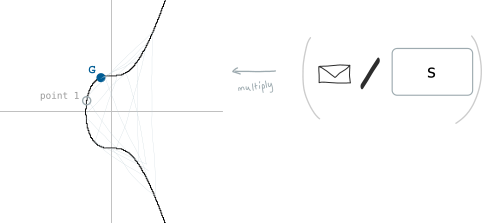### Point 2

Divide `r` by `s`. The second point is just the public key multiplied by this value: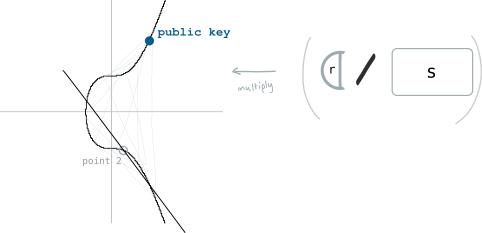### Finally…

Now if we add these two points together, we will get a third point on the curve: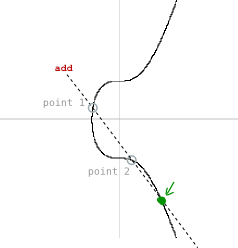And if the x-coordinate of this third point is the same as the x-coordinate of the random point we started with (`r`), then this is proof that the digital signature was created using the private key connected to this public key.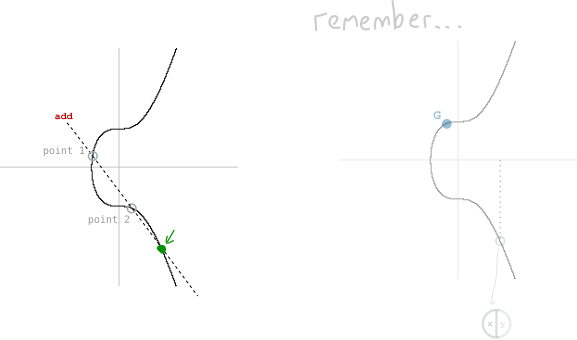• ECDSA - Technical details on how to sign transactions in Bitcoin.

## Resources

Hey there, it's Greg.

I'll let you know about cool website updates, or if something seriously interesting happens in bitcoin.

Don't worry, it doesn't happen very often.